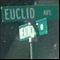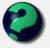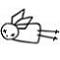Discussion about math, puzzles, games and fun.   Useful symbols: ÷ × ½ √ ∞ ≠ ≤ ≥ ≈ ⇒ ± ∈ Δ θ ∴ ∑ ∫  π  -¹ ² ³ °

You are not logged in.

## #1 2006-12-24 15:17:08

Ricky
ModeratorRegistered: 2005-12-04
Posts: 3,791

### Inequalities

I was shopping for some Christmas presents in Borders (a book store) and I of course stumbled my way into the math section.  Found a really cheap and interesting book on inequalities.

One of the earlier problems is:

Prove that the square root of two lies in between m/n and (m+2n) / (m + n), for all values of m, n in the natural numbers.

First off, I didn't believe it till I tried a few numbers out.  Then I was shocked at how simple the proof was.  If anyone wants to try it out, go ahead and do so.  Else, I will post the proof in a few days.

"In the real world, this would be a problem.  But in mathematics, we can just define a place where this problem doesn't exist.  So we'll go ahead and do that now..."

Offline

## #2 2006-12-24 17:56:20

MathsIsFunRegistered: 2005-01-21
Posts: 7,696

### Re: Inequalities

"In Between" meaning that:

if m/n < √2 then √2 < (m+2n) / (m + n)

if m/n > √2 then √2 > (m+2n) / (m + n)

I get that by random calculations. For example:

3/2 = 1.5, (3+2×2)/(3+2) = 1.4

Wow!

"The physicists defer only to mathematicians, and the mathematicians defer only to God ..."  - Leon M. Lederman

Offline

## #3 2006-12-25 04:05:20

Ricky
ModeratorRegistered: 2005-12-04
Posts: 3,791

### Re: Inequalities

I had exactly the same reaction.

"In the real world, this would be a problem.  But in mathematics, we can just define a place where this problem doesn't exist.  So we'll go ahead and do that now..."

Offline

## #4 2006-12-25 05:54:17

Zhylliolom
Real MemberRegistered: 2005-09-05
Posts: 412

### Re: Inequalities

My phraseology may not be the best here, but you get the idea. The proof is easy indeed, I could do it in my head (which is my excuse for not having it as polished as possible here).

It is obvious that for any given natural numbers m and n, either

or

is true, since √2 is irrational and therefore cannot be equal to a rational number, and thus the result follows from trichotomy.

Now consider the inequality

where the ? denotes the unknown direction of the inequality. Since m and n are natural numbers, their sum is nonzero and positive, and thus we may multiply each side my m + n without reversing the inequality:

Once again, n is a natural number and thus is nonzero and positive, so we may divide each side by n and maintain the direction of the inequality:

Now subtract m/n and √2 from each side to obtain

which gives (after dividing through by 1 - √2, flipping the inequality, rationalizing, then flipping again so that we now have the original direction (these steps are left to the reader as an exercise))

which gives the direction of the inequality as the opposite of the "initial condition" m/n ¿ √2, where ¿ denotes the original (and opposite) inequality direction, so that if m/n < √2 then (m + 2n)/(m + n) > √2 or if m/n > √2 then (m + 2n)/(m + n) < √2.

Thus, either

or

is true for natural numbers m and n.

Offline

## #5 2006-12-25 10:45:59

MathsIsFunRegistered: 2005-01-21
Posts: 7,696

### Re: Inequalities

Nice Proof.

Now, I *think* it may also be true that the square root of 3 lies in between m/n and (m+3n) / (m + n), and so on.

"The physicists defer only to mathematicians, and the mathematicians defer only to God ..."  - Leon M. Lederman

Offline

## #6 2006-12-26 03:09:19

mathsyperson
ModeratorRegistered: 2005-06-22
Posts: 4,900

### Re: Inequalities

I tried following through Zhylliolom's method with k instead of 2, and I'm pretty sure you're right.

For values such as 4, 9, 16, etc., Zhylliolom's first step (saying either m/n > √k or m/n < √k) breaks down.
However, if such a value is chosen, and m and n are chosen such that m/n = √k, then we can still use Zhylliolom's method to show that (m+kn)/(m+k) will also equal √k, so your corollary still holds.

Very interesting problem though, Ricky. What was that book called?

Why did the vector cross the road?
It wanted to be normal.

Offline

## #7 2006-12-26 11:29:25

MathsIsFunRegistered: 2005-01-21
Posts: 7,696

### Re: Inequalities

Ah yes, if √k is rational, then there is an m/n that is equal. But otherwise the "between" works.

I wonder if there is a generalization for cube roots etc ...

"The physicists defer only to mathematicians, and the mathematicians defer only to God ..."  - Leon M. Lederman

Offline

## #8 2006-12-26 16:56:33

Ricky
ModeratorRegistered: 2005-12-04
Posts: 3,791

### Re: Inequalities

Very interesting problem though, Ricky. What was that book called?

The book is called "Analytic Inequalities", by Nicholas D. Kazarinoff.  It's aim is to fill a gap left between algebraic (high school) math and analysis, specifically that of inequalities.  Analysis is just full of them, and many students don't have a lot of experience with them.  I was one of those.  I did just find in my introduction to analysis course, however did struggle a lot with inequalities.

I will use this thread to post some of the interesting problems I find in it (or ones I have difficulty with).

Problem 1.2

Show that:

There is a very crucial hint given, but I will wait a day or two to post it.  Note that no computer nor calculator may be used.

"In the real world, this would be a problem.  But in mathematics, we can just define a place where this problem doesn't exist.  So we'll go ahead and do that now..."

Offline

## #9 2006-12-26 21:53:58

Patrick
Real MemberRegistered: 2006-02-24
Posts: 1,005

### Re: Inequalities

Analytic Inequalities at Amazon.com. This looks like a very interresting book. Might buy itSupport MathsIsFun.com by clicking on the banners.
What music do I listen to? Clicky click

Offline

## #10 2006-12-28 14:05:54

Ricky
ModeratorRegistered: 2005-12-04
Posts: 3,791

### Re: Inequalities

First prove that:

As with most proofs on the natural numbers, the recommended way to proceed is by induction.

"In the real world, this would be a problem.  But in mathematics, we can just define a place where this problem doesn't exist.  So we'll go ahead and do that now..."

Offline

## #11 2007-01-24 17:02:00

John E. Franklin
MemberRegistered: 2005-08-29
Posts: 3,588

### Re: Inequalities

I can't prove it, but it seems right, since x^2 + x + (x+1) = (x +1)^2
I know that from playing around on a calculator, but sorry I can't prove it yet.

igloo myrtilles fourmis

Offline

## #12 2007-01-25 05:37:16

Ricky
ModeratorRegistered: 2005-12-04
Posts: 3,791

### Re: Inequalities

We know that:

So:

and so:

Thus:

And so:

which means that:

And so:

Whew!  The other side is exactly the same, just start off with the fact that:

"In the real world, this would be a problem.  But in mathematics, we can just define a place where this problem doesn't exist.  So we'll go ahead and do that now..."

Offline